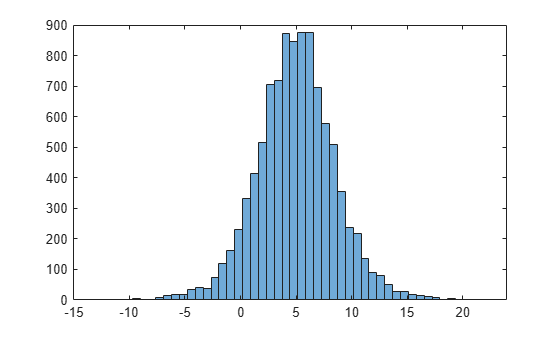Documentation

LoglogisticDistribution

Loglogistic probability distribution object

Description

A LoglogisticDistribution object consists of parameters, a model description, and sample data for a loglogistic probability distribution.

The loglogistic distribution is closely related to the logistic distribution. If x is distributed loglogistically with parameters μ and σ, then log(x) is distributed logistically with mean and standard deviation. This distribution is often used in survival analysis to model events that experience an initial rate increase, followed by a rate decrease.

The loglogistic distribution uses the following parameters.

ParameterDescriptionSupport
muMean of logarithmic values$\mu >0$
sigmaScale parameter of logarithmic values$\sigma >0$

Creation

There are several ways to create a LoglogisticDistribution probability distribution object.

• Create a distribution with specified parameter values using makedist.

• Fit a distribution to data using fitdist.

• Interactively fit a distribution to data using the Distribution Fitter app.

Properties

expand all

Distribution Parameters

Mean of logarithmic values for the loglogistic distribution, specified as a positive scalar value.

Data Types: single | double

Scale parameter of logarithmic values for the loglogistic distribution, specified as a positive scalar value.

Data Types: single | double

Distribution Characteristics

This property is read-only.

Logical flag for truncated distribution, specified as a logical value. If IsTruncated equals 0, the distribution is not truncated. If IsTruncated equals 1, the distribution is truncated.

Data Types: logical

This property is read-only.

Number of parameters for the probability distribution, specified as a positive integer value.

Data Types: double

This property is read-only.

Covariance matrix of the parameter estimates, specified as a p-by-p matrix, where p is the number of parameters in the distribution. The (i,j) element is the covariance between the estimates of the ith parameter and the jth parameter. The (i,i) element is the estimated variance of the ith parameter. If parameter i is fixed rather than estimated by fitting the distribution to data, then the (i,i) elements of the covariance matrix are 0.

Data Types: double

This property is read-only.

Logical flag for fixed parameters, specified as an array of logical values. If 0, the corresponding parameter in the ParameterNames array is not fixed. If 1, the corresponding parameter in the ParameterNames array is fixed.

Data Types: logical

This property is read-only.

Distribution parameter values, specified as a vector.

Data Types: single | double

This property is read-only.

Truncation interval for the probability distribution, specified as a vector containing the lower and upper truncation boundaries.

Data Types: single | double

Other Object Properties

This property is read-only.

Probability distribution name, specified as a character vector.

Data Types: char

This property is read-only.

Data used for distribution fitting, specified as a structure containing the following:

• data: Data vector used for distribution fitting.

• cens: Censoring vector, or empty if none.

• freq: Frequency vector, or empty if none.

Data Types: struct

This property is read-only.

Distribution parameter descriptions, specified as a cell array of character vectors. Each cell contains a short description of one distribution parameter.

Data Types: char

This property is read-only.

Distribution parameter names, specified as a cell array of character vectors.

Data Types: char

Object Functions

 cdf Cumulative distribution function icdf Inverse cumulative distribution function iqr Interquartile range mean Mean of probability distribution median Median of probability distribution negloglik Negative loglikelihood of probability distribution paramci Confidence intervals for probability distribution parameters pdf Probability density function proflik Profile likelihood function for probability distribution random Random numbers std Standard deviation of probability distribution truncate Truncate probability distribution object var Variance of probability distribution

Examples

collapse all

Create a loglogistic distribution object using the default parameter values.

pd = makedist('Loglogistic')
pd =
LoglogisticDistribution

Log-Logistic distribution
mu = 0
sigma = 1

Create a loglogistic distribution object by specifying the parameter values.

pd = makedist('Loglogistic','mu',5,'sigma',2)
pd =
LoglogisticDistribution

Log-Logistic distribution
mu = 5
sigma = 2

Generate random numbers from the loglogistic distribution and compute their log values.

rng(19) % for reproducibility
x = random(pd,10000,1);
logx = log(x);

Compute the mean of the log values.

m = mean(logx)
m = 4.9828

The mean of the log of x is equal to the mu parameter of x, since x has a loglogistic distribution.

Plot logx.

histogram(logx,50)The plot shows that the log values of x have a logistic distribution.